# NIUHE

## Generative and discriminative models

Generative (joint) models $P(c, d)$

• Model the distribution of individual classes and place probabilities over both observed data and hidden variables (such as labels)
• E.g. n-gram models, hidden Markov models, probabilistic context-free grammars, IBM machine translation models, Naive Bayes...

Discriminative (conditional) models $P(c|d)$

• Learn boundaries between classes. Take data as given and put probability over the hidden structure give the data.
• E.g. logistic regression, maximum entropy models, conditional random fields, support-vector machines...

## Naive Bayes classifier

Bayes' Rule: $P(c|d) = \frac{P(c)P(d|c)}{P(d)}$ This estimates the probility of document $d$ being in class $c$, assuming document length $n_d$ and tokens $t$: $P(c|d) = P(c)P(d|c) = P(c)\prod_{1 ≤i≤n_d}P(t_i|c)$ Independence Assumptions

Note that we assume $P(t_i|c) = P(t_j|c)$ independent of token position. This is the naive part of Naive Bayes.

The best class is the maximum a posteriori (MAP) clss: $c_{map} = \arg\max_{c\in C}P(c|d) = \arg\max_{c\in C}P(c)\prod_{1≤i≤n_d}P(t_i|c)$ Multiplying tons of small probabilities is tricky, so log space it: $c_{map} = \arg\max_{c\in C}(\log P(c) + \sum_{1≤i≤n_d}\log P(t_i|c))$ Finally: zero probabilities are bad. Add smoothing: $P(t|c) = \frac{T_{ct}}{\sum_{t'\in V}T_{ct'}} => P(t|c) = \frac{T_{ct} + 1}{\sum_{t'\in V}T_{ct'}+|V|}$ This is Laplace or add-1 smoothing.

• Simple
• Interpretable
• Fast (linear in size of training set and test document)
• Text representation trivial (bag of words)

Drawbacks

• Independence assumptions often too strong
• Sentence/document structure not taken into account
• Naive classifier has zero probabilities; smoothing is awkward

Naive Bayes is a generative model!!! $P(c|d)P(d) = P(d|c)P(c) = P(d, c)$ While we are using a conditional probability $P(c|d)$ for classification, we model the joint probability of $c$ and $d$.

This meas it is trivial to invert the process and generate new text given a class label.

## Feature Representations

A feature representation (of text) can be viewed as a vector where each element indicates the presence or absence of a given feature in a document.

Note: features can be binary (presence/absence), multinomial (count) or continuous (eg. TF-IDF weighted).

## Logistic Regression

A general framework for learning $P(c|d)$ is logistic regression

• logistic : because is uses a logistic function
• regression : combines a feature vector ($d$) with weights ($\beta$) to compute an answer

Binary case: $P(true|d) = \frac{1}{1 + \exp(\beta_0 + \sum_i\beta_iX_i)}$

$P(false|d) = \frac{\exp(\beta_0 + \sum_i\beta_iX_i)}{1 + \exp(\beta_0+\sum_i\beta_iX_i)}$

Multinomial case: $P(c|d) = \frac{\exp(\beta_{c,0} + \sum_i\beta_{c,i}X_i)}{\sum_{c'}\exp(\beta_{c',0} + \sum_i\beta_{c',i}X_i)}$ The binary and general functions for the logistic regression can be simplified as follows: $P(c|d) = \frac{1}{1+\exp(-z)}$

$P(c|d) = \frac{\exp(z_c)}{\sum_{c'}\exp(z_{c'})}$

which are referred to as the logistic and softmax function.

Given this model formulation, we want to learn parameters $β$ that maximise the conditional likelihood of the data according to the model.

Due to the softmax function we not only construct a classifier, but learn probability distributions over classifications.

There are many ways to chose weights $β$:

• Perceptron Find misclassified examples and move weights in the direction of their correct class
• Margin-Based Methods such as Support Vector Machines can be used for learning weights
• Logistic Regression Directly maximise the conditional log-likelihood via gradient descent.

• Still reasonably simple
• Results are very interpretable
• Do not assume statistical independence between features!

Drawbacks

• Harder to learn than Naive Bayes
• Manually designing features can be expensive
• Will not necessarily generalise well due to hand-craftedfeatures

## Representing Text with a RNN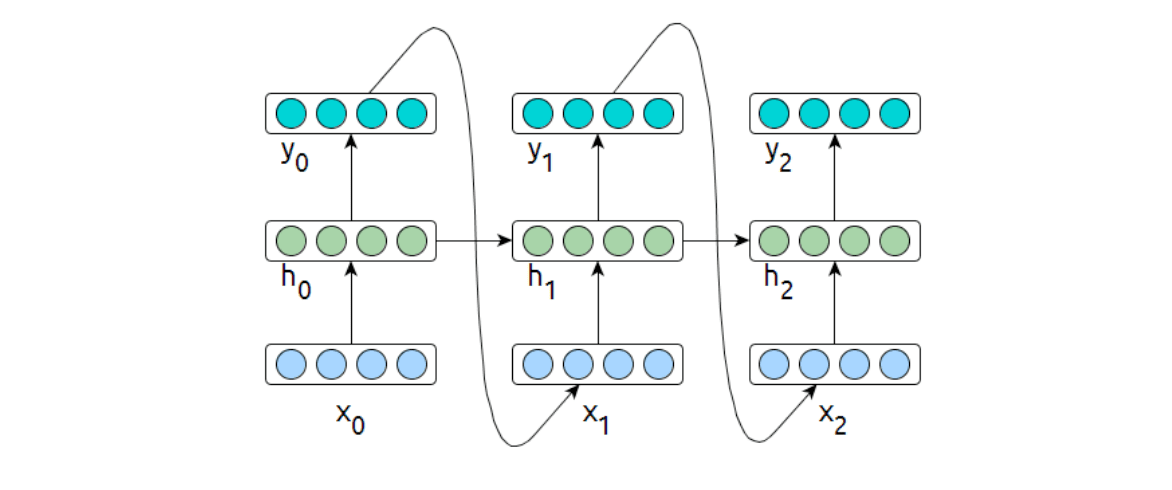• $h_i$ is a function of $x\{0:i\}$ and $h\{0:i−1\}$
• It contains information about all text read up to point $i$.
• The first half of this lecture was focused on learning a representation $X$ for a given text

So in order to classify text we can simply take a trained language model and extract text representations from the final hidden state $c_n$.

Classification as before using a logistic regression: $P(c|d) = \frac{\exp(\beta_{c,0} + \sum_i\beta_{c,i}h_{ni})}{\sum_{c'}\exp(\beta_{c',0} + \sum_i\beta_{c',i}h_{ni})}$ ✅ Can use RNN + Logistic Regression out of the box ✅ Can in fact use any other classifier on top of $h$ ! ❌ How to ensure that $h$ pays attention to relevant aspects of data?

Move the classification function inside the network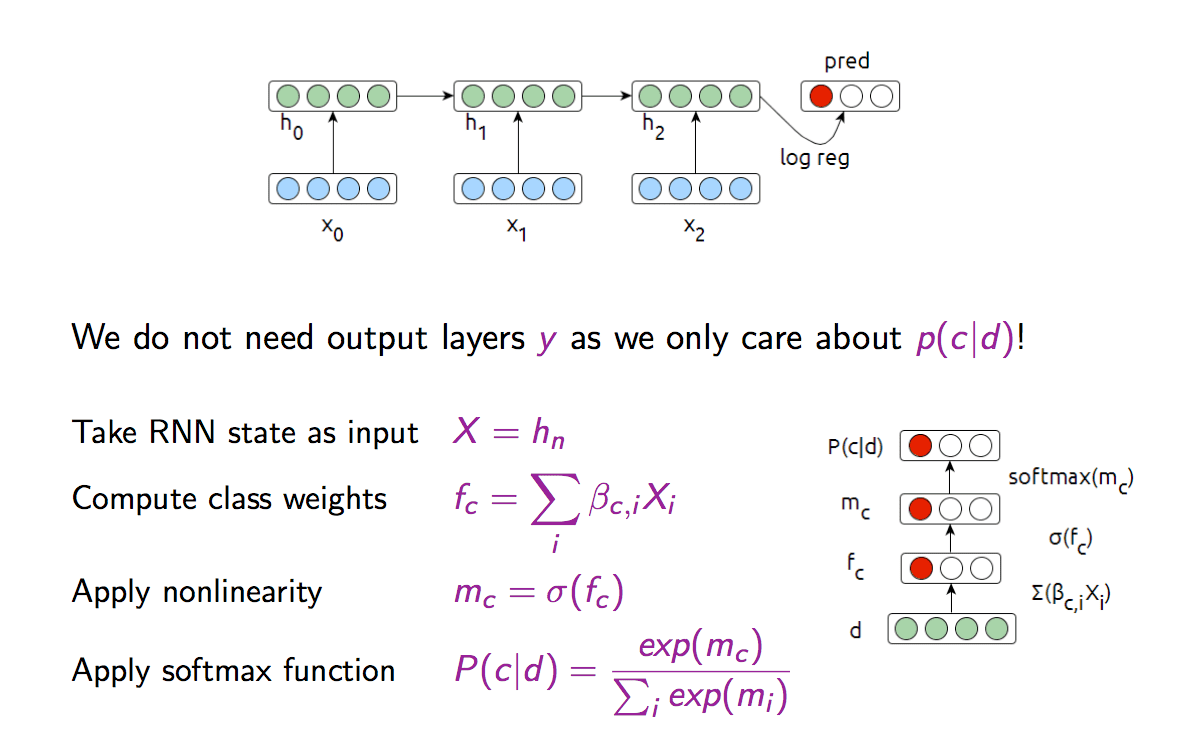This is a simple Multilayer Perceptron (MLP). We can train the model using the cross-entropy loss: $L_i = -\sum_c y_c \log P(c|d_i) = -\log (\frac{\exp(m_c)}{\sum_j\exp(m_j)})$

• Cross-entropy is designed to deal with errors on probabilities.
• Optimizing means minimizing the cross-entropy between the estiated class probabilities ($P(c|d)$) and the ture distribution.
• There are many alternative losses (hinge-loss, square error, L1 loss).

Dual Objective RNN

In practice it may make sense to combine an LM objective with classifier training and to optimise the two losses jointly.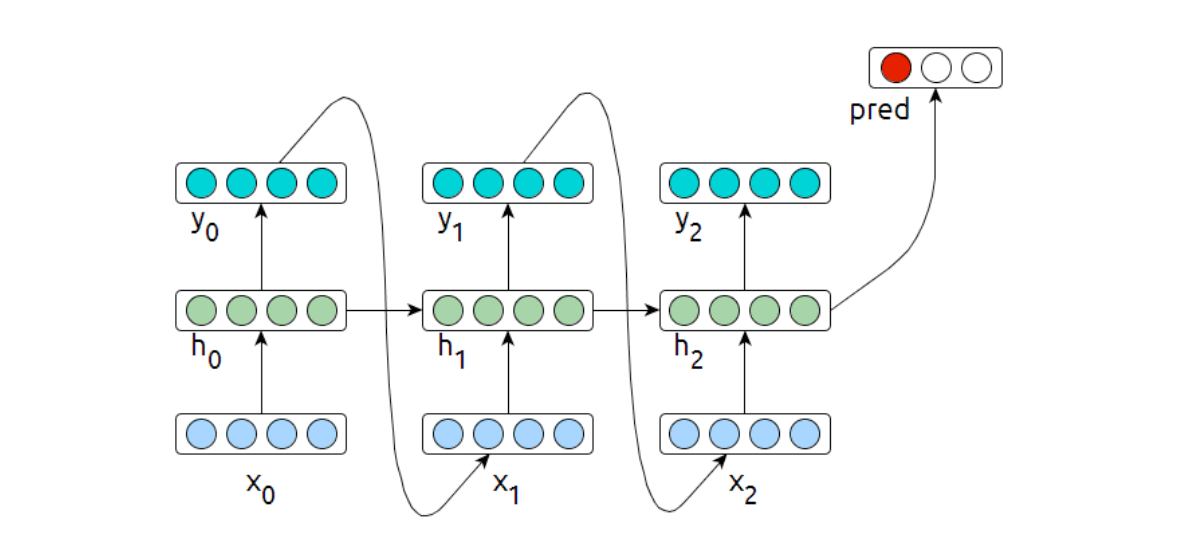$J = \alpha J_{class} + (1-\alpha)J_{lm}$ Such a joint loss enables making use of text beyond labelled data.

Bi-Directional RNNs

Another way to add signal is to process the input text both in a forward and in a backward sequence.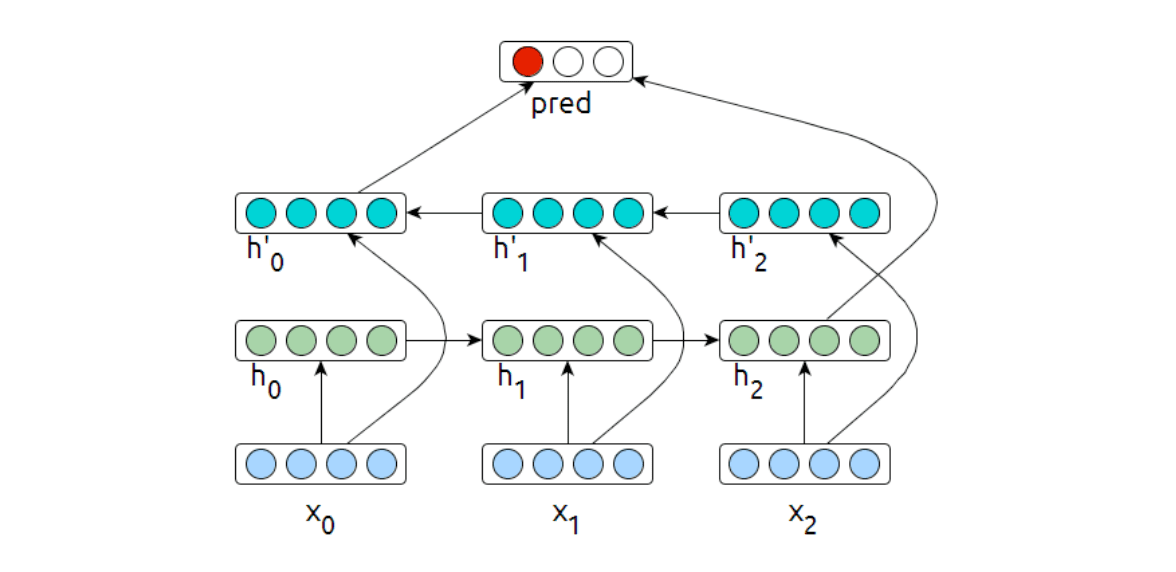The update rules for this directly follow the regular forward-facing RNN arhitecture. In practice, bidirectional networks have shown to be more robust than unidirectional networks.

A bidirectional network can be used as a classifier simply by redefining $d$ to be the concatenation of both final hidden states: $d = (\rightarrow{h_n}||h_0\leftarrow)$ RNN Classifier can be either a generative or a discriminative model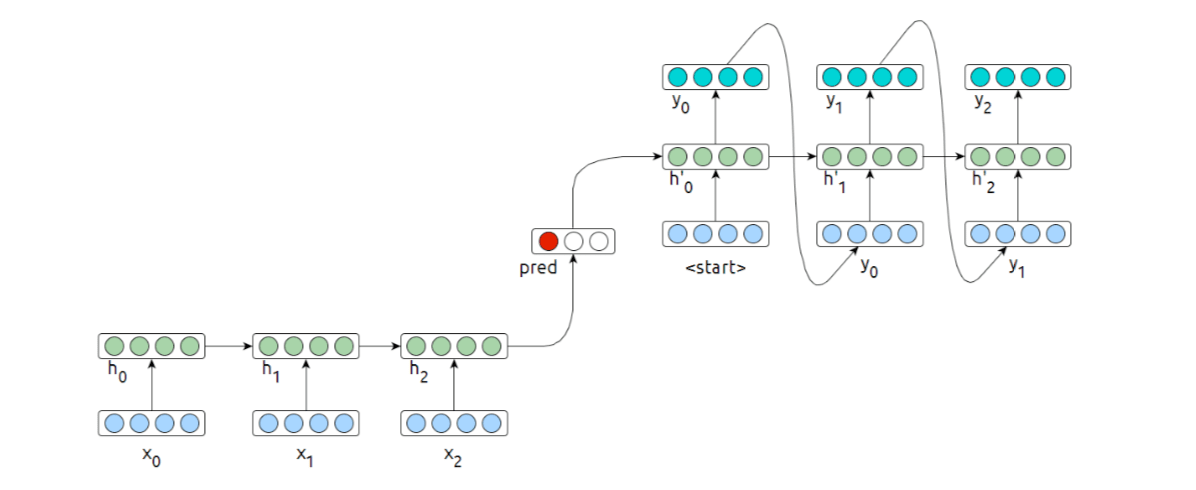Encoder: discriminative (it does not model the probability of the text) Joint-model: generative (learns both $P(c)$ and $P(d)$).

## Convolutional Neural Network

Reasons to consider CNNs for Text:

• ✅ Really fast (GPU)
• ✅ BOW is often sufficient
• ✅ Actually can take some structure into account
• ❌ Not sequential in its processing of input data
• ❌ Easier to discriminate than to generate variably sized data